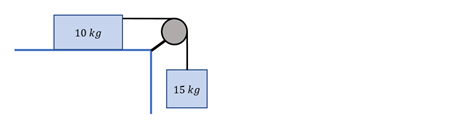# Problem: In the following figure, the pulley doesn’t rotate without friction, so it limits how fast the system can move. In this particular case, the 15 kg mass can only drop at a maximum speed of 10 m/s. At this speed, how much work does the pulley need to do every meter the 15 kg mass drops?

###### Problem Details

In the following figure, the pulley doesn’t rotate without friction, so it limits how fast the system can move. In this particular case, the 15 kg mass can only drop at a maximum speed of 10 m/s. At this speed, how much work does the pulley need to do every meter the 15 kg mass drops?